# Universe

(diff) ← Older revision | Latest revision (diff) | Newer revision → (diff)

A setwhich is closed under the formation of unions, singletons, subelements, power sets, and pairs; more precisely:

1)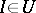,implies;

2)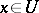implies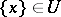;

3)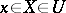implies;

4)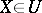implies;

5)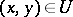if and only if.

The existence of infinite universes in axiomatic set theory is equivalent to the existence of strongly inaccessible cardinals (cf. Cardinal number). A universe is a model for Zermelo–Fraenkel set theory. Universes were introduced by A. Grothendieck in the context of category theory in order to introduce the "set" of natural transformations of functors between (-) categories, and in order to admit other "large" category-theoretic constructions.

How to Cite This Entry:
Universe. Encyclopedia of Mathematics. URL: http://encyclopediaofmath.org/index.php?title=Universe&oldid=11866
This article was adapted from an original article by B. Pareigis (originator), which appeared in Encyclopedia of Mathematics - ISBN 1402006098. See original article